Try out our new practice tests completely free!# Quiz 2: Diodes and ApplicationsThe anode of a diode is usually marked by a band, a tab, or some other feature.
(True False)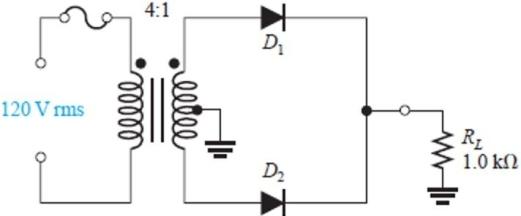Figure 1 -For the circuit in Figure 1, assume ideal diodes. The peak inverse voltage is approximately
(Multiple Choice)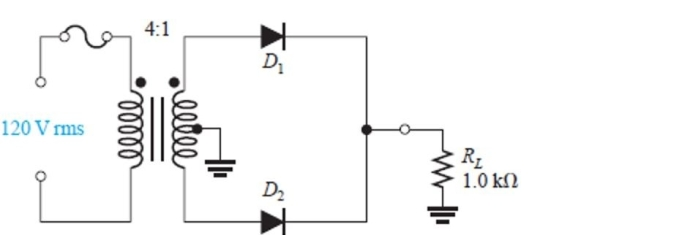Figure 1 -For the circuit in Figure 1, assume ideal diodes. The average voltage you should observe across RL is
(Multiple Choice)To forward bias a diode, the n- region is connected to the positive side of a voltage source.
(True False)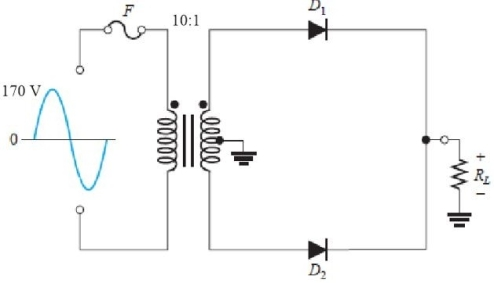Figure 3 -For the circuit in Figure 3, assume practical diodes. The peak output voltage across the load is
(Multiple Choice)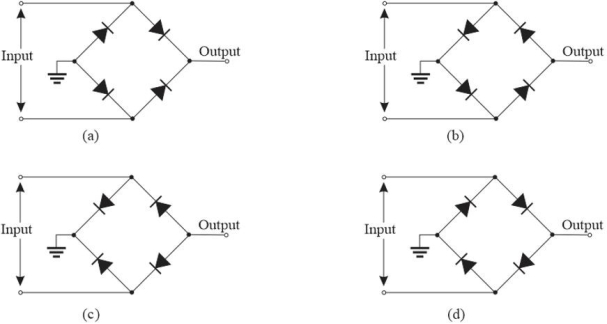Figure 2 -Refer to Figure 2. The circuit that will produce a negative output is
(Multiple Choice)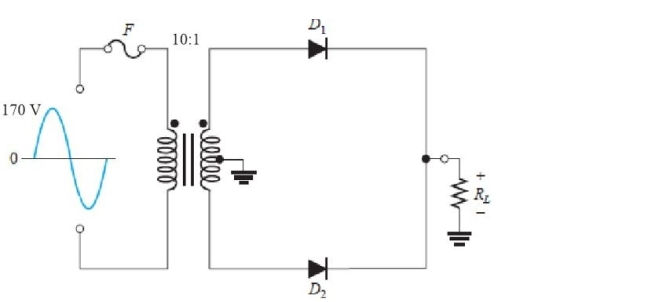Figure 3 -For the circuit in Figure 3, assume a diode opens. As a result, the peak output voltage will be
(Multiple Choice)An ideal diode is considered to have a small forward voltage drop across the junction.
(True False)When a diode is forward- biased, the depletion region narrows.
(True False)A silicon diode measures a very high value of resistance in one direction and very low resistance in the other. This indicates the diode is probably
(Multiple Choice)The component(s)immediately preceding the voltage regulation stage in a power supply is
(Multiple Choice)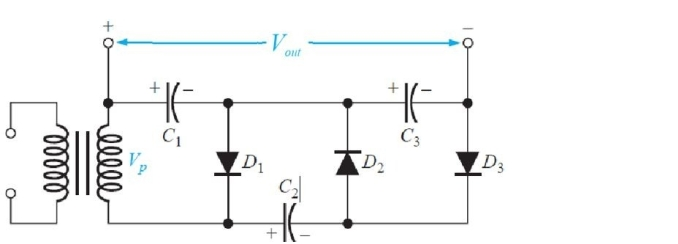Figure 9 -The circuit in Figure 9 is a
(Multiple Choice)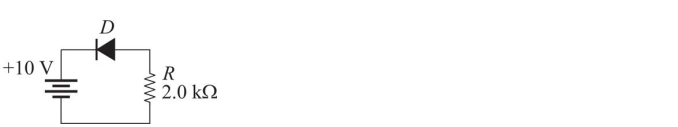Figure 4 -Refer to Figure 4. Assume the diode is a practical diode. The current in the diode will be
(Multiple Choice)C:\User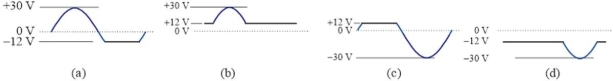Figure 7 For questions 34, 35, and 36, assume ideal diodes -Which circuit in Figure 6 will produce the waveform in Figure 7(a)?
(Multiple Choice)When a diode is reverse- biased, the current is normally extremely small.
(True False)Assume an ideal diode has a series 10 k▲ resistor and is forward- biased with a 20 V source. The current in the diode is
(Multiple Choice)For a forward- biased diode, as temperature increases, forward current also increases.
(True False)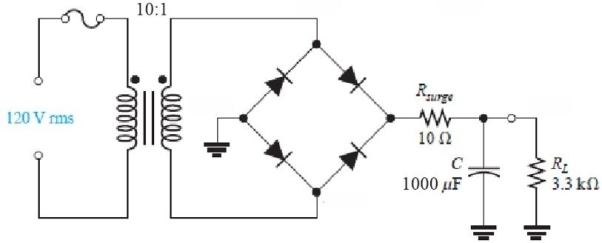Figure 5 -Refer to the circuit in Figure 5. The current in the load resistor is approximately
(Multiple Choice)Dynamic resistance is the same as linear resistance.
(True False)C:\UserFigure 7 For questions 34, 35, and 36, assume ideal diodes -Which circuit in Figure 6 will produce the waveform in Figure 7(b)?
(Multiple Choice)## Filters

• Essay(0)
• Multiple Choice(0)